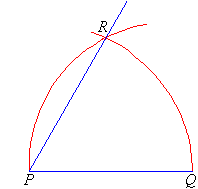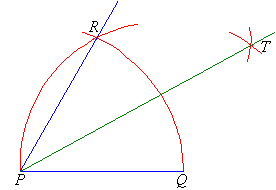# ANGLES – TRIANGLES [CONTD]

Welcome to Class !!

In today’s Basic Technology class, We will continue with our class on Angles. We hope you enjoy the class!

## Constructing a 60º Angle

We know that the angles in an equilateral triangle are all 60º in size.  This suggests that to construct a 60º angle we need to construct an equilateral triangle as described below.

Step 1:  Draw the arm PQ.

Step 2:  Place the point of the compass at P and draw an arc that passes through Q.
Step 3:  Place the point of the compass at Q and draw an arc that passes through P.  Let this arc cut the arc drawn in Step 2 at R### Constructing a 30º Angle

We know that:

So, to construct an angle of 30º,

first, construct a 60º angle and then bisect it. Often, we apply the following steps.

Step 1:  Draw the arm PQ.

Step 2:  Place the point of the compass at P and draw an arc that passes through Q.
Step 3:  Place the point of the compass at Q and draw an arc that cuts the arc drawn in Step 2 at R.

Step 4:  With the point of the compass still at Q, draw an arc near T as shown.
Step 5:  With the point of the compass at R, draw an arc to cut the arc drawn in Step 4 at T.
Step 6:  Join T to P.  The angle QPT is 30º.### Constructing a 90º Angle

We can construct a 90º angle either by bisecting a straight angle or using the following steps.

Step 1:  Draw the arm PA.

Step 2:  Place the point of the compass at P and draw an arc that cuts the arm at Q.
Step 3:  Place the point of the compass at Q and draw an arc of radius PQ that cuts the arc drawn in Step 2 at R.
Step 4:  With the point of the compass at R, draw an arc of radius PQ to cut the arc drawn in Step 2 at S.
Step 5:  With the point of the compass still at R, draw another arc of radius PQ near T as shown.
Step 6:  With the point of the compass at S, draw an arc of radius PQ to cut the arc drawn in step 5 at T.
Step 7:  Join T to P. The angle APT is 90º.We have come to the end of this class. We do hope you enjoyed the class?

Should you have any further question, feel free to ask in the comment section below and trust us to respond as soon as possible.

In our next class, we will be talking about Circle. We are very much eager to meet you there.

Are you a Parent? Share your quick opinion and win free 2-month Premium Subscription

Don`t copy text!